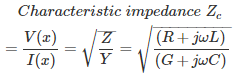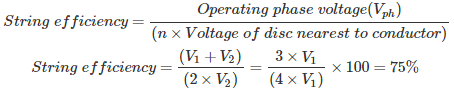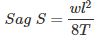# MCQs on Power Systems

##### Page 16 of 67. Go to page 1 2 3 4 5 6 7 8 9 10 11 12 13 14 15 16 17 18 19 20 21 22 23 24 25 26 27 28 29 30 31 32 33 34 35 36 37 38 39 40 41 42 43 44 45 46 47 48 49 50 51 52 53 54 55 56 57 58 59 60 61 62 63 64 65 66 67
01․ Pin insulators are used up to
11 kV
33 kV
120 kV
any voltage level

Overhead line insulators: 1. Pin insulators used up to 33 kV. 2. Suspension type insulators all voltages 33 kV to 765 kV. 3. Strain insulators are used in river/ road/ railway crossings and dead end end tower to reduce sag. Pin type insulators are used only up to 33 kV. Beyond this voltage more insulation material is required, which increases cost of the insulation. At higher voltage suspension or string insulators are used.

02․ Find the number of insulator discs required in string insulator, if operating voltage is 220 kV and maximum voltage of each disc is 11 kV?
20
10
13
21

Number of insulator discs n = ( Operating voltage/phase)/ Maximum voltage of each disc Operating voltage/phase = 220*10³/√3 = 127 kV Maximum voltage of each disc = 11 kV Number of insulator discs n = 127/11 =11.547 ≈ 12 For safety operation one extra disc is added to the string. Therefore, total number of insulator discs = 13

03․ In a 3-phase transmission line, each phase is supported by 3 insulated discs, maximum voltage of each disc is 20 kV. Find the operating voltage of the transmission line per phase if efficiency of string is 80.6%.
48.36 kV
52.84 kV
83.76 kV
20 kV

String efficiency = Operating phase voltage(Vph)/(n * Voltage of disc nearest to conductor) Where, n = number of discs Given that, efficiency = 0.806 Maximum voltage of each disc = 20 kV Therefore, 0.806 = Vph/(3*20*10³) Operating phase voltage(Vph) = 48.36 kV

04․ A cable has inductance of 0.22 mH per km and capacitance of 0.202 µF per km. The surge impedance of the cable is
28 Ω
33 Ω
40 Ω
52 Ω

Characteristic impedance (Zc): It will give the relation between voltage and current flowing through the transmission line which are depending on the transmission line parameters, i.e., R,G,L and C.For loss less line. R = G = 0 Surge impedance Zs = √(L/C) Zs = √(0.22×10^-3/(0.202×10^-6)) Zs = 33 Ω

05․ Which of the following decides the insulation level of 400 kV, EHV line?
lighting voltage
skin effect
switching over voltage

At transmission line voltages up to 230 kV the insulation level is decided by the requirement of protection against lightning. In EHV, switching surges cause higher over voltages than lightning and therefore mainly responsible for insulation level decision.

06․ Two insulator discs of identical capacitance value C make up a string for a 22 kV, 50 Hz, single phase overhead line insulation system. If pin to earth capacitance is also C, then find the string efficiency?
75%
90%
50%
86%%Where, n = number of discs Voltage of disc nearest to conductor V2 = V1(1+K) Where, K = Pin to earth capacitance/Capacitance = C/C =1 V2 = 2V1 % String efficiency = 75%

07․ Which of the following insulator is practically used for railway crossings?
pin insulator
string insulator
strain insulator
all of the above

Overhead line insulators: 1. Pin insulators used up to 33 kV. 2. Suspension type insulators all voltages 33 kV to 765 kV. 3. Strain insulators are used in river/ road/ railway crossings and dead end end tower to reduce sag. Strain insulator is one of the suspension insulator is used to reduce sag in the transmission lines connected between the two towers and it is practically used in railway crossings, road crossings, river crossings and in the dead end towers.

08․ Which of the following statements is/are true? By increasing of temperature,
sag increase
tension decrease
both 1 and 2
neither 1 nor 2Where, l = length of the conductor w = weight of the conductor T = tension By increasing the temperature, the length of the conductor will increase, this will increases the sag. As the sag is inversely proportional to tension. So, as sag increases, tension will decrease.

09․ Stock bridge dampers are used to
increase the sag
reduce the vibrations at the point of support
reduce the tension
all of the above

Due to unequal ice loading vibrations are produced in the conductors. If these vibrations are extended up to the point of support of the conductor, bolts and nuts are loosen, finally producing fault in the system. Therefore, to reduce these vibrations at the point of support, vibration or stock bridge dampers are used.

10․ String chart is drawn between
temperature (T) on X- axis, sag(S) and tension (T) on Y-axis
T on Y-axis and S and T on on X-axis
both 1 and 2
nether 1 nor 2

String chart is a graph drawn between sag and tension on Y- axis verses temperature of region on X-axis. By using the string chart the height of the tower and tension to be maintained on the conductor is calculated for maintaining optimum value of sag in the conductor.

<<<1415161718>>>